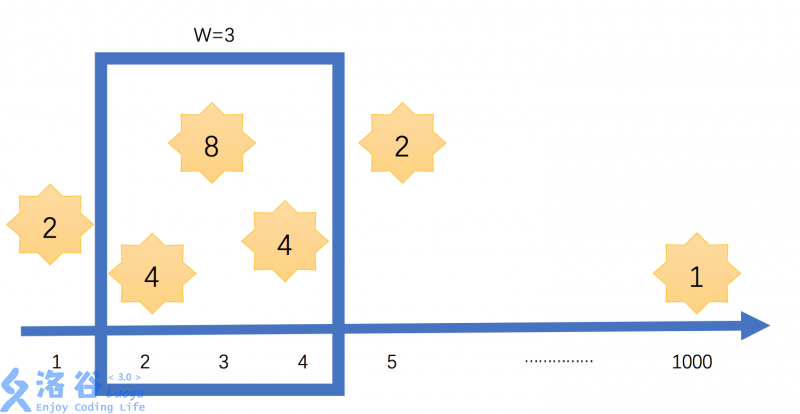6 3
1 2
2 4
3 8
4 4
5 2
1000 1

16

### 说明/提示### 题解代码

``看到这个题目不禁想起我的经历。那个梦中的她，或许早已成为，生死边界的彼岸花。直到入土也难以相见。她或许，早已化作一场梦，一场永无现实的梦 。 "在你窗外闪耀的星星"可能只是一道掠影，浮光一现 消逝，如泪。 */ include using namespace std;long long int a,b;long long int ans;void qsort(int x,int y){    int i=x,j=y;    int mid=b[(x+y)/2];    do    {        while(b[i]mid)         j--;        if(i<=j)         {             swap(a[i],a[j]);             swap(b[i],b[j]);             i++;j--;         }     }while(i<=j);     if(x>n>>m;    for(int i=1;i<=n;i++)     cin>>b[i]>>a[i];    qsort(1,n);         int j=1;    long long s=0;    for(int i=1;i<=n;i++)    {        s-=a[i-1];              while(j<=n and b[j]-b[i]<m)        {            s=a[j]+s;            j++;        }        ans=max(ans,s);    }    cout<<ans<<endl;    return 0;}//纵然千秋已逝//愿你//芳华初始``